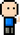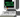# Josh Meets Computer# Levenstein Algorithm

June 19, 2018

This algorithm deals with finding the edit distance between two strings. This is a classic algorithm that represents the power of Dynamic Programming, where solving a problem with regular recursion will result in repeated computation of identical sub problems. In contrast, dynamic programming allows us to record the solution to the subproblems of a recursion and look them up instead of re-calculating.

### Approach

The function receives two strings as arguments. First thing it does is set the longer string to be string #1 (perhaps a personal preference). It uses the length of the two strings to create an empty matrix of (string 1) rows and (string 2) columns, with an extra row and column for null values. From there it calculates the minimum number of inserts, removals and replacements needed to be made to turn s1[i..m] into s2[i…n] by checking for the minimum value between the left, top and top left adjacent values in the created matrix.

``````def edit_distance1(str1, str2):

if len(str1) < len(str2):
str1, str2 = str2, str1

m = len(str1)
n = len(str2)

dp = [[0 for x in range(n + 1) ] for x in range(m + 1)]

for i in range(m + 1):
for j in range(n + 1):
if i == 0:
dp[i][j] = j  # while str1 character is null, you have to remove j letters

elif j == 0:
dp[i][j] = i  #while str2 character is null, you have to remove i letters

elif str1[i-1] == str2[j-1]:
dp[i][j] = dp[i-1][j-1]  # if two characters match, the cost is same as previous value

else:  # pick the lowest cost option to get the strings to match
dp[i][j] = 1 + min(
dp[i][j-1],    # insert a character
dp[i-1][j],    # Remove a character
dp[i-1][j-1])  # Replace a character

return dp[m][n]

if __name__ == "__main__":
print('')
print('Necessary edits:',edit_distance1('love', 'Jovial'))``````
``Necessary edits: 4``